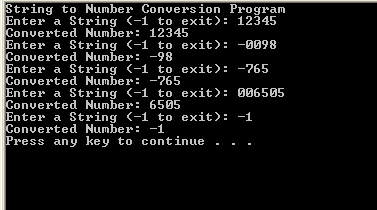# Turbo C++ - String to Number Conversion Program

Here is the Turbo C++ source code for string to number conversion.

First the length of the string is identified. To handle the negative numbers, the first character is being checked for '-'. If it is '-', then negative number indication flag is set to true (1).

The next character ascii value is being deducted from character '0' to get the numeric value. If it is in between 0-9, then it will be stored into the result and when the subsequent character is getting in, the previous value is multiplied by 10. otherwise a non numeric character is found and the return value would be zero.

Example String: 5647

Result Value

1st Iteration: 5

2nd Iteration: 5 * 10 + 6 = 56

3rd Iteration: 56 * 10 + 5 = 564

4th Iteration: 564 * 10 + 7 = 5647

## Source Code

#include <stdio.h>

#include <string.h>

#include <iostream.h>

int StringToNumber(const char *buffer)

{

int result = 0;

int startIndex = 0;

int negativeNumber = 0;

if(buffer == '-')

{

negativeNumber = 1;

startIndex = 1;

}

for(int i = startIndex; i < strlen(buffer); i++)

{

if(buffer[i] >= '0' && buffer[i] <= '9')

{

int digit = buffer[i] - '0';

result = result * 10 + digit;

}

else

return 0;

}

if(negativeNumber == 1)

result *= -1;

return result;

}

int main()

{

char buffer;

std::cout << "String to Number Conversion Program\n";

while(1)

{

std::cout << "Enter a String (-1 to exit): ";

std::cin >> buffer;

int number = StringToNumber(buffer);

std::cout << "Converted Number: " << number << "\n";

if(number == -1)

break;

}

return 0;

}

## Output